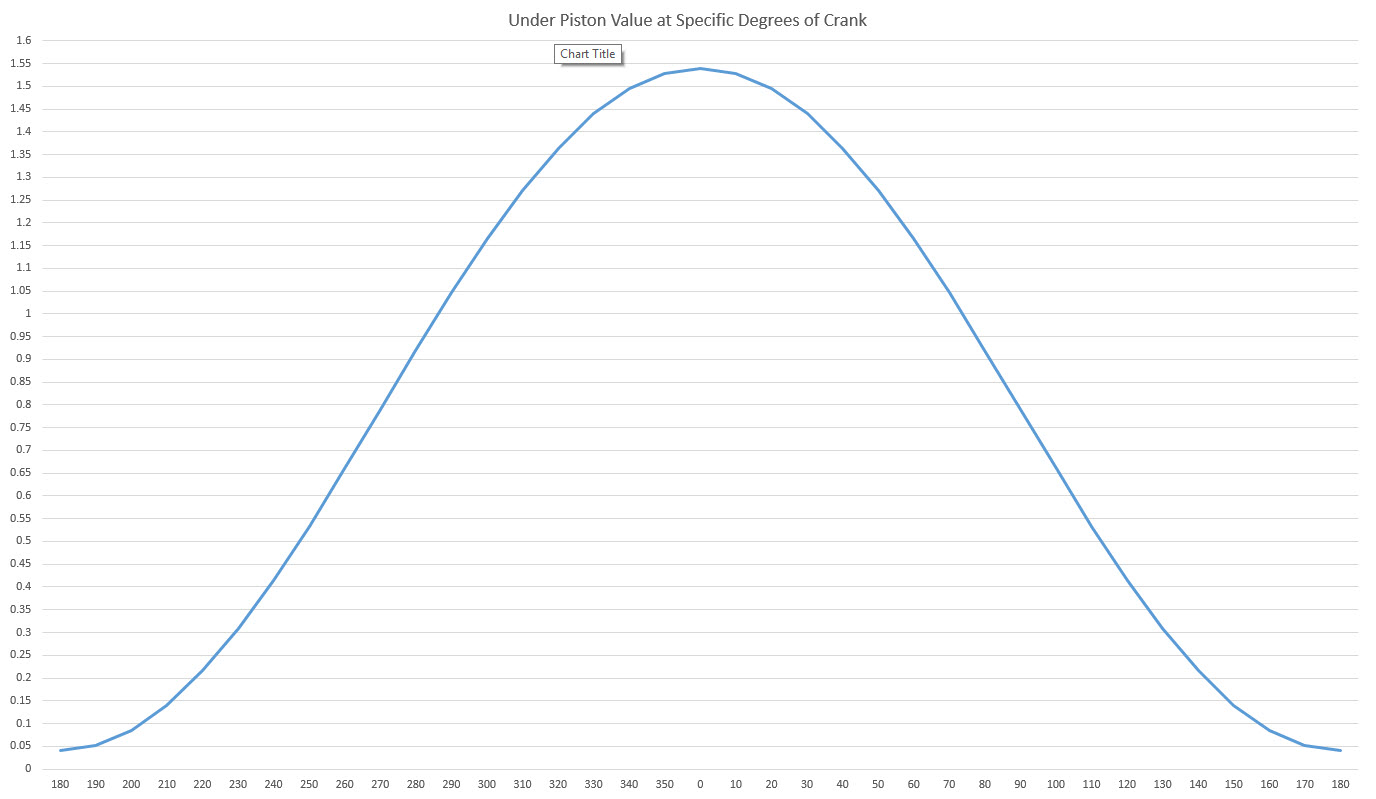# Top Stop Distances

## Bourke Engine 101

### Top Stop Distances

#### Graphical DiagramPosition In Degrees Distance from the Top of the Piston to the Bottom of the Head in Inches
TDC = 180 0.0400″
BTDC = 175, ATDC = 185 0.0429″
BTDC = 170, ATDC = 190 0.0513″
BTDC = 165, ATDC = 195 0.0656″
BTDC = 160, ATDC = 200 0.0853″
BTDC = 157.5, ATDC = 202.5 0.0970″ *
BTDC = 155, ATDC = 205 0.1106″
BTDC = 150, ATDC = 210 0.1403″
BTDC = 140, ATDC = 220 0.2155″
BTDC = 135, ATDC = 225 0.2592″ #
BTDC = 130, ATDC = 230 0.3078″
BTDC = 120, ATDC = 240 0.4149″
BTDC = 112.5, ATDC = 247.5 0.5025″ #
BTDC = 110, ATDC = 250 0.5333″
BTDC = 100, ATDC = 260 0.6597″
BTDC = 90, ATDC = 270 0.7900″ #
BTDC = 80, ATDC = 280 0.9202″
BTDC = 70, ATDC = 290 1.0466″
BTDC = 67.5, ATDC = 292.5 1.0769″ #
BTDC = 60, ATDC = 300 1.1651″
BTDC = 50, ATDC = 310 1.2720″
BTDC = 45, ATDC = 315 1.3203″ #
BTDC = 40, ATDC = 320 1.3644″
BTDC = 30, ATDC = 330 1.4395″
BTDC = 22.5, ATDC = 337.5 1.4830″ #
BTDC = 20, ATDC = 340 1.4948″
BTDC = 15, ATDC = 345 1.5145″
BTDC = 10, ATDC = 350 1.5285″
BTDC = 5, ATDC = 355 1.5371″
Bottom Dead Center = 0/360 1.5400″

* Because of the geometry (refined Scottish Yorke/reverse roller cam), from 157.5 degrees BTDC to 180 degrees TDC (22.5 degrees of crankshaft travel), the piston only moves up 0.057″ (top stop). Likewise, from 180 degrees TDC to 202.5 degrees ATDC (22.5 degrees of crankshift travel), the piston only moves down 0.057″ (top stop). The piston is at or near top dead center for 45° of crankshaft travel.

# After the crankshaft reaches a point of mechanical advantage (22.5 degrees ATDC), the piston/saddle/rod/yoke plate assembly is shot (like a projectile) towards the opposite cylinder. The distance the piston travels for every 22.5 degrees of crankshaft travel is shown below:

• 202.5 degrees ATDC to 225 degrees ATDC = 0.1622″
• 225 degrees ATDC to 247.5 degrees ATDC = 0.2433″
• 247.5 degrees ATDC to 270 degrees ATDC = 0.2875″
• 270 degrees ATDC to 292.5 degrees ATDC = 0.2869″
• 292.5 degrees ATDC to 315 degrees ATDC = 0.2434″
• 315 degrees ATDC to 337.5 degrees ATDC = 0.1627″